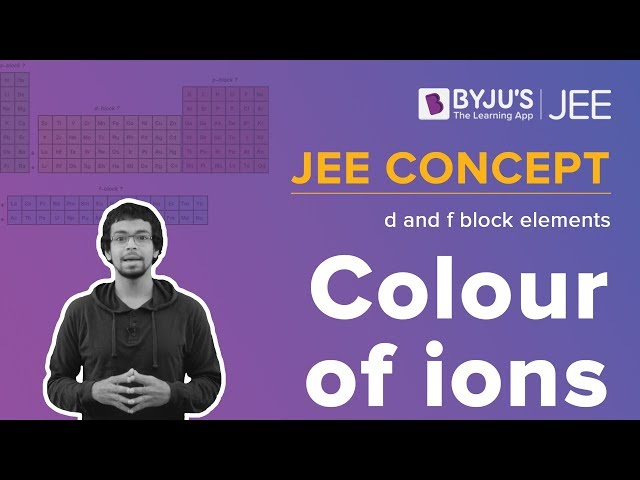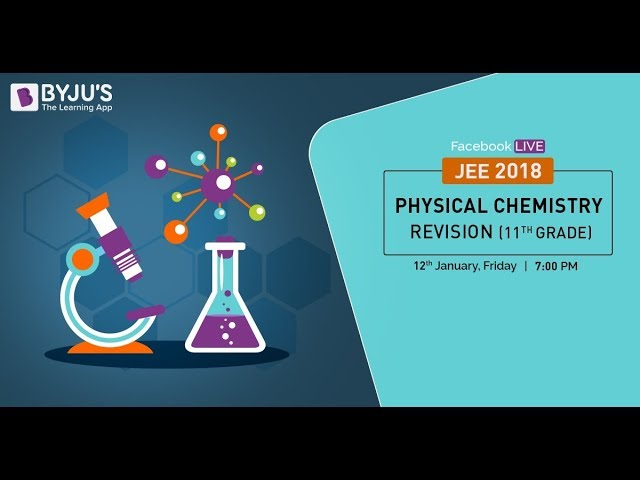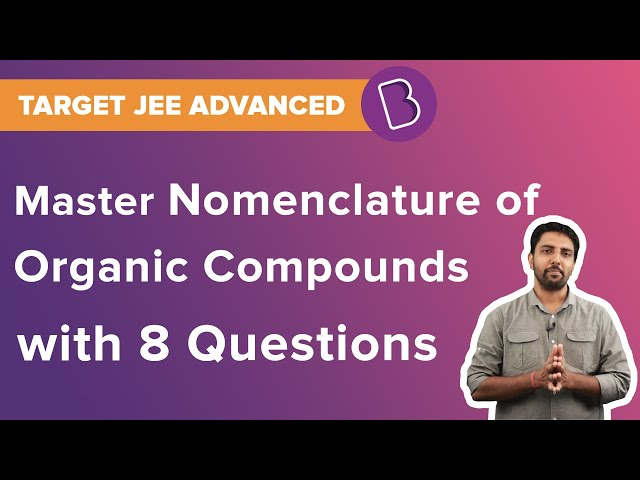Win up to 100% scholarship on Aakash BYJU'S JEE/NEET courses with ABNAT Win up to 100% scholarship on Aakash BYJU'S JEE/NEET courses with ABNAT

# JEE Main 2020 Chemistry Paper With Solutions Sept 6 (Shift 2)

JEE Main 2020 Paper with Solutions Chemistry – Shift 2 (Sept 6) are available here. Set of solved papers will help the candidate understand the key features of the exam. Students are advised to go through the previous year papers for JEE Main in order to develop a strong and effective preparation strategy. Doing so will help candidates to familiarize themselves with all the pattern of the paper as well as the type of questions likely to arise. Download Chemistry paper to practise offline.
September 6 Shift 2 – Chemistry

Question 1: Match the following :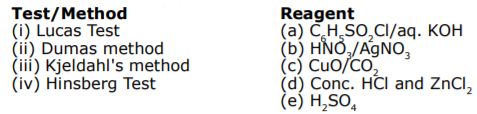1) (i)-(d),(ii)-(c),(iii)-(e),(iv)-(a)
2) (i)-(b),(ii)-(a),(iii)-(c),(iv)-(d)
3) (i)-(b),(ii)-(d),(iii)-(e),(iv)-(a)
4) (i)-(d),(ii)-(c),(iii)-(b)-(iv)-(e)

By Theory

Question 2: The IUPAC name of the following compound is: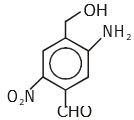1) 2-nitro-4-hydroxymethyl-5-amino benzaldehyde
2) 3-amino-4-hydroxymethyl-5-nitro benzaldehyde
3) 4-amino-2-formyl-5-hydroxymehtyl nitrobenzene
4) 5-amino-4-hydroxymethyl-2-nitro benzaldehyde

Question 3: For the given cell;

Cu(s)|Cu2+(C1M)||Cu2+(C2M)|Cu(s)

Change in Gibbs energy (ΔG) is negative, if :

1) C2 = √2 C1
2) C2 = C1/√2
3) C1 = 2C2
4) C2 = C1

E = 0 – (0.059/2) log[C1/C2]

ΔG = -nFE

= +nF x RT/nF x 2.303 log[C1/C2]

log[C1/C2] < 0

C1 < C2

C2 = √2 C1

Question 4: Reaction of an inorganic sulphite X with dilute H2SO4 generates compound Y. Reaction of Y with NaOH gives X. Further, the reaction of X with Y and water affords compound Z. Y and Z, respectively, are :

1) SO2 and NaHSO3
2) S and Na2SO3
3) SO2 and Na2SO3
4) SO3 and NaHSO3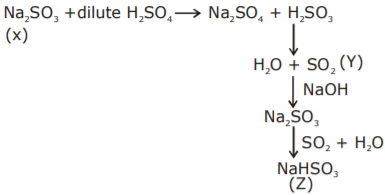So, Y= SO2 and Z = NaHSO3

Question 5: The value of KC is 64 at 800 K for the reaction N2(g) + 3H2(g)

$$\begin{array}{l}\rightleftharpoons\end{array}$$
2NH3(g)

The value of KC for the following reaction is :

NH3 (g)

$$\begin{array}{l}\rightleftharpoons\end{array}$$
(1/2) N2(g) + (3/2) H2 (g)

1) 1/4
2) 8
3) 1/8
4) 1/64

Kc = (64)-1/2

Kc = 1/8

Question 6: The correct match between Item – I (Starting material) and Item – II (reagent) for the preparation of benzaldehyde is :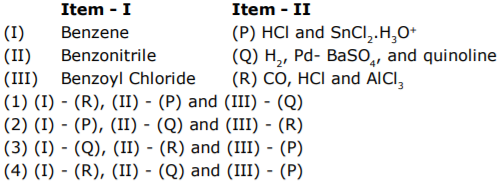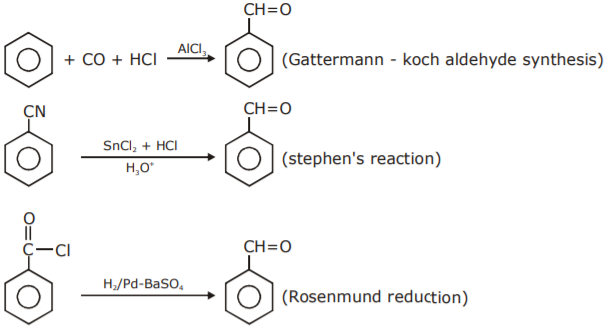Question 7: For a d4 metal ion in an octahedral field, the correct electronic configuration is :

1) e2g t22g when Δ0 < P
2) t42g eog when Δ0 < P
3) t32g e1g when Δ0 > P
4) e1g t32g when Δ0 < P

If Δ<sub>o</sub> < P the electronic configuration for d<sup>4</sup> configuration will be

$$\begin{array}{l}t_{2g}^{3} e_{g}^{1}\end{array}$$
.

Question 8: The correct match between Item – I and Item – II is :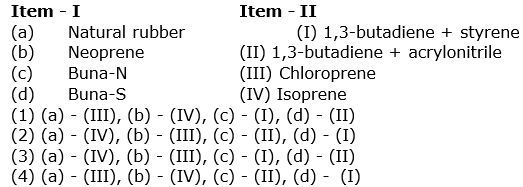By Theory

Question 9: Which one of the following statement is not true?

1) Lactose contains α -glycosidic linkage between C1 of galactose and C4 of glucose.
2) Lactose is a reducing sugar and it gives Fehling’s test.
3) On acid hydrolysis, lactose gives one molecule of D(+)-glucose and one molecule of D(+)-galactose.
4) Lactose(C11H22O11) is a disaccharide and it contains 8 hydroxyl groups.

Lactose contains β-glycosidic linkage between C1 of galactose and C4 of glucose.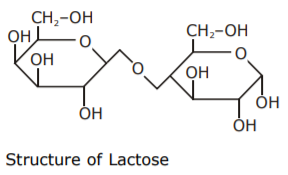Question 10: The element that can be refined by distillation is :

1) tin
2) gallium
3) zinc
4) nickel

Zinc -> Refined by distillation

Question 11: Match the following compounds (Column-I) with their uses (Column-II) :

 S. No. Column – I S. No. Column – II (I) Ca(OH)2 (A) Casts of statues (II) NaCl (B) White wash (III) CaSO4 . (1/2) H20 (C) Antacid (IV) CaCO3 (D) Washing soda preparation

1) (I)-(B),(II)-(C),(III)-(D),(IV)-(A)
2) (I)-(C),(II)-(D),(III)-(B),(IV)-(A)
3) (I)-(B),(II)-(D),(III)-(A),(IV)-(C)
4) (I)-(D),(II)-(A),(III)-(C),(IV)-(B)

(i) Ca(OH)2 -> used in white wash due to its disinfectant nature

(ii) CaCO3 -> it used as an Antacid

(iii) CaSO4 . (1/2)H2O -> For making casts of statues and busts

(iii) Preparation of washing soda (sodium carbonate)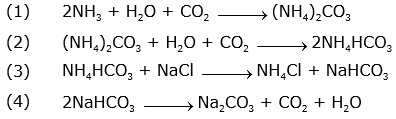Question 12: Mischmetal is an alloy consisting mainly of :

1) lanthanoid and actinoid metals
2) lanthanoid metals
3) actinoid metals
4) actinoid and transition metals

Misch metal – well known alloy is mischmetal which consists of Lanthanoid metal

(~ 95%) and iron (~ 5%) and Traces of S, C, Ca and Al

Question 13: For a reaction, 4M(s) + n O2(g) -> 2 M2 On (s) the free energy change is plotted as a function of temperature. The temperature below which the oxide is stable could be inferred from the plot as the point at which :

1) the free energy change shows a change from negative to positive value.
2) the slope changes from positive to zero
3) the slope changes from positive to negative.
4) the slope changes from negative to positive.

ΔG = ΔH – TΔS

ΔG = –ve (stable oxide)

ΔG = +ve (unstable oxide)

Question 14: The increasing order of the boiling points of the major products A,B and C of the following reaction will be :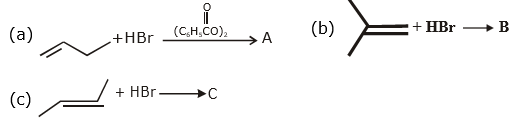1) A < B < C
2) C < A < B
3) A < C < B
4) B < C < A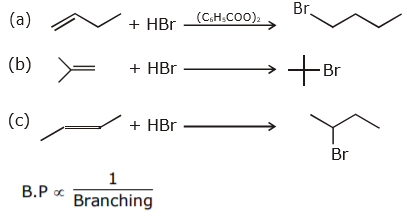Question 15: The average molar mass of chlorine is 35.5g mol-1. The ratio of 35Cl to 37Cl in naturally occurring chlorine is close to :

1) 1 : 1
2) 3 : 1
3) 2 : 1
4) 4 : 1

[35x + 37y]/[x+y] = 35.5

1.5y = –0.5x

x/y = 3/1

Question 16: Which of the following compound can be prepared in good yield by Gabriel phthalimide synthesis?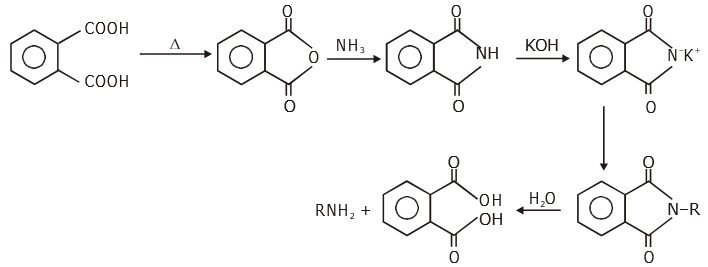In this reaction, the alkyl halide should be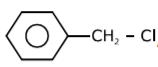, which can gives SN2 reaction easily.

Question 17: The reaction of NO with N2O4 at 250 K gives:
1) N2O
2) NO2
3) N2O5
4) N2O3

NO + N2O4

$$\begin{array}{l}\overset{250k}{\rightarrow}\end{array}$$
N2O3

Question 18: A set of solution is prepared using 180 g of water as a solvent and 10g of different nonvolatile solutes A, B and C. The relative lowering of vapour pressure in the presence of these solutes are in the order [Given, molar mass of A = 100 g mol-1 ; B = 200g mol-1 ; C = 10,000g mol-1]

1) A > C > B
2) B > C > A
3) C > B > A
4) A > B > C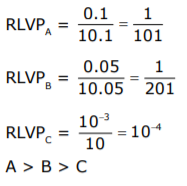Question 19: Dihydrogen of high purity (> 99.95%) is obtained through :

1) the electrolysis of acidified water using Pt electrodes.
2) the reaction of Zn with dilute HCl
3) the electrolysis of brine solution
4) the electrolysis of warm Ba(OH)2

High purity (>99.95%) dihydrogen is obtained by electrolysing warm aqueous barium hydroxide solution between nickel electrodes.

Question 20: A crystal is made up of metal iron ‘M1‘ and ‘M2‘ and oxide ions. Oxide ions form a ccp lattice structure. The cation ‘M1‘ occupies 50% of octahedral voids and the cation ‘M2‘ occupies 12.5% of tetrahedral voids of oxide lattice. The oxidation number of ‘M1’ and ‘M2’ are, respectively:

1) +2, +4
2) +3, +1
3) +4, +2
4) +1, +3

(M1)2 (M2)1 O4

+2x + y = 8

x = +2 and y = +4

Question 21: For Freundlich adsorption isotherm, a plot of log (x/m) (y-axis) and log p (x-axis) gives a straight line. The intercept and slope for the line is 0.4771 and 2, respectively. The mass of gas, adsorbed per gram of adsorbent if the initial pressure is 0.04 atm, is…………….×10-4g. (log 3=0.4771)

x/m = KP1/n

Log(x/m) = Log(K) + (1/n) log (P)

K = 3 x 1/n = 2

n = ½

x/m = 3P1/n

x/m = 3 x (4 x 10-2)2 = 48 x 10-4 g

Question 22: The atomic number of Unnilunium is …………….

Fact

Question 23: A solution of phenol in chloroform when treated with aqueous NaOH gives compound P as a major product. The mass percentage of carbon in P is ……………. (to the nearest integer)

(Atomic mass : C = 12; H = 1; O = 16)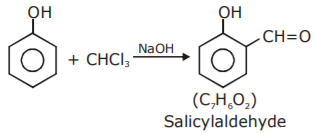mass of Salicylaldehyde = 12 × 7 + 6 × 1 + 16 × 2 = 122

mass of carbon = 12 × 7 = 84

The mass % of carbon = 84/122 x 100 = 68.85%

Question 24: The rate of a reaction decreased by 3.555 times when the temperature was changed from 40oC to 30oC. The activation energy (in KJ mol-1) of the reaction is……… [Take; R = 8.314 J mol-1 K-1 In 3.555 = 1.268]

K40oC = K ; K30oC = K/3.555

ln{3.555} = Ea/R {1/303 – 1/313}

Ea = [1.268 x 8.314 x 313 x 303]/10

Ea = 99980.7 J/mol.

Ea = 99.98 kJ/mol.

Ea = 100 kJ/mol.

Question 25: If the solubility product of AB2 is 3.20 × 10-11 M3, then the solubility of AB2 in pure water is ……….× 10-4 mol L-1 [Assuming that neither kind of ion reacts with water].

AB2(s)

$$\begin{array}{l}\rightleftharpoons\end{array}$$
A+2aq + 2Baq

S 2S

Ksp = 4s3 = 32 x 10-12

S = 2 x 10-4 mol/lit

## Video Lessons – September 6 Shift 2 Chemistry